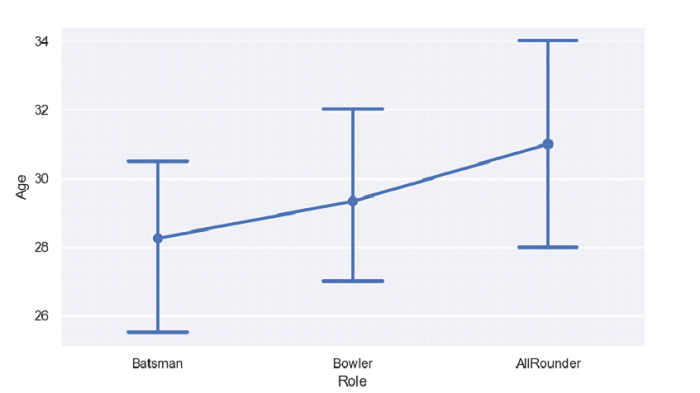# Python Pandas - Draw a point plot and set a cap to the error bars with Seaborn

PythonServer Side ProgrammingProgramming

Point Plot in Seaborn is used to show point estimates and confidence intervals using scatter plot glyphs. The seaborn.pointplot() is used for this. Set caps to the error bars using the capsize parameter.

Let’s say the following is our dataset in the form of a CSV file − Cricketers.csv

At first, import the required libraries −

import seaborn as sb
import pandas as pd
import matplotlib.pyplot as plt

Load data from a CSV file into a Pandas DataFrame −

dataFrame = pd.read_csv("C:\\Users\\amit_\\Desktop\\Cricketers.csv")


Setting caps to the error bars using the capsize parameter −

sb.pointplot(dataFrame['Role'],dataFrame['Age'], capsize=.3)

## Example

Following is the code −

import seaborn as sb
import pandas as pd
import matplotlib.pyplot as plt

# Load data from a CSV file into a Pandas DataFrame

sb.set_theme(style="darkgrid")

# point plot
# setting caps to the error bars using the capsize parameter
sb.pointplot(dataFrame['Role'],dataFrame['Age'], capsize=.3)

# display
plt.show()

## Output

This will produce the following output −